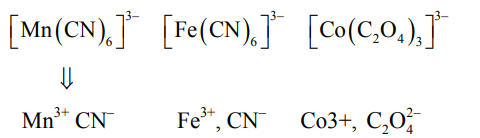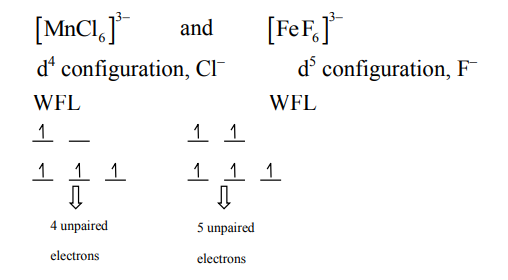# Given below are two statements :Question:

Given below are two statements :

Statement I : $\left[\mathrm{Mn}(\mathrm{CN})_{6}\right]^{3-},\left[\mathrm{Fe}(\mathrm{CN})_{6}\right]^{3-}$ and $\left[\mathrm{Co}\left(\mathrm{C}_{2} \mathrm{O}_{4}\right)_{3}\right]^{3-}$ are d $^{2} \mathrm{sp}^{3}$ hybridised.

Statement II : $\left[\mathrm{MnCl}_{6}\right]^{3-}$ and $\left[\mathrm{FeF}_{6}\right]^{3-}$ are paramagnetic and have 4 and 5 unpaired electrons, respectively.

In the light of the above statements, choose the correct answer from the options given below :

1. Statement I is correct but statement II is false

2. Both statement I and statement II are false

3. Statement I is incorrect but statement II is true

4. Both statement I and statement II are are true

Correct Option: , 4

Solution:$\mathrm{d}^{4}$ configuration, SFL $\mathrm{d}^{5}$ configuration, SFL $\mathrm{d}^{6}$ configuration, Chelating ligand

$\Rightarrow$ All will have larger splitting hence $\mathrm{d}^{2} \mathrm{sp}^{3}$ hybridisation